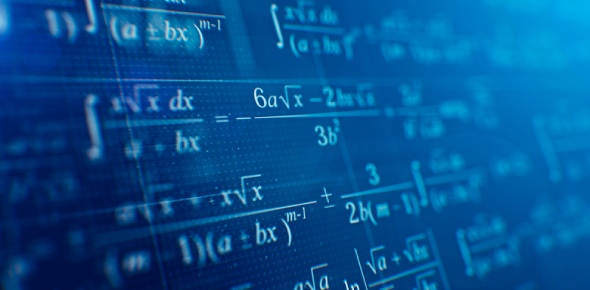# Mathematics Exam: MCQ! Trivia Quiz

10 Questions | Total Attempts: 67SettingsRelated Topics
• 1.
What are the factors of  8x2 - 13x + 5?
• A.

(8x - 5) and (x - 1)

• B.

(2x - 5) and (4x - 1)

• C.

(8x + 1) and (x - 5)

• D.

(2x - 1) and (4x - 5)

• E.

( 4x-1) and (2x-5)

• 2.
An aquarium is a closed box with a length of 50 cm, a width of 40 cm, and a height of 30 cm. Find the surface area of this aquarium.
• A.

18 800 cm2

• B.

60 000 cm2

• C.

4700 cm2

• D.

9400 cm2

• E.

8400 cm2

• 3.
A plane contains atleast three non-collinear points and space contains atleast four non-coplanar points.
• A.

True

• B.

False

• 4.
The formula for the area of the square is A=(s)*(s)
• A.

True

• B.

False

• 5.
The ____________ of the triangle is greater than each of its remote interior angles.
• 6.
Progression series:  what is the next digit - 1,2,6,42,1806,_____.
• 7.
The difference between mathematical equation to mathematical expression is?
• A.

Inequality sign

• B.

Equal sign

• C.

• D.

Subtraction sugn

• 8.
The parts of polygons are/is.
• A.

Apex

• B.

Vertex

• C.

Side

• D.

Reterior angles

• E.

Angles

• F.

Diaghonals